# Hausdorff gap

(diff) ← Older revision | Latest revision (diff) | Newer revision → (diff)

Ifandare subsets of, then one writesprovided that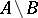is finite. In addition,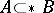means that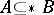while, moreover,is infinite. Finally,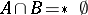means thatis finite.

Letandbe infinite cardinal numbers (cf. also Cardinal number), and consider the following statement: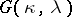: There are a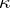-sequenceof subsets ofand a-sequence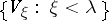of subsets ofsuch that:

1)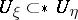if;

2)if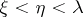;

3) if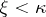and, then;

4) there does not exist a subsetofsuch that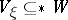for allandfor all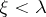.

In [a2], F. Hausdorff proved that) is false while) is true. The sets that witness the fact that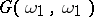) holds are called a Hausdorff gap. K. Kunen has shown in [a3] that it is consistent with Martin's axiom (cf. also Suslin hypothesis) and the negation of the continuum hypothesis that) and) both are false. Here,is the cardinality of the continuum (cf. also Continuum, cardinality of the). He also proved that it is consistent with Martin's axiom and the negation of the continuum hypothesis that) and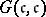) both are true. See [a1] for more details.

How to Cite This Entry:
Hausdorff gap. Encyclopedia of Mathematics. URL: http://encyclopediaofmath.org/index.php?title=Hausdorff_gap&oldid=13106
This article was adapted from an original article by J. van Mill (originator), which appeared in Encyclopedia of Mathematics - ISBN 1402006098. See original article